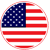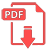# Home | Mathematics | Geometry(Planimetry)

## Pythagorean Triples

Pythagoras was a mathematician born in Greece in about 570 BC. He was interested in mathematics, science and philosophy. He is known to most people because of the Pythagoras Theorem that is about a property of all triangles with a right-angle (an angle of 90&deg;): legs a,b; hyp=h If a triangle has one angle which is a right-angle (i.e. 90&deg;) then there is a special relationship between the lengths of its three sides:

If the longest side (called the hypotenuse) is h and the other two sides (next to the right angle) are called a and b, then:

a2 + b2 = h2

Pythagoras' Theorem

The square of the longest side is the same as the sum of the squares of the other two sides.

h2 = a2 + b2
is only true for right-angled triangles.English